Range is explained as difference between maximum and minimum observation of given data. If Xm denotes maximum observation Xo that denotes minimum observation then this range is defined as:

Range = Xm –X0

For grouped data, the range is known as difference between upper boundary of highest class and also lower boundary of lowest class. It also helps to calculate by making use of difference between mid points of highest class and lowest class. It is called as simplest measure of dispersion.

It offers general idea about complete spread of observations. Application and utility in quality control method can also be used to maintain any quality of products.

Applications of range

The range can also be an effective way to get through basic understanding of how to spread out the data. This is quite easy to calculate as it needs only basic arithmetic operation. There is also availability of other application of range.

Through range it is possible to estimate any other measure of spread, standard deviation. A complicated formula helps to find standard deviation which is called range rule.

We may define range to eliminate any outliers and also extreme points in data set. In inter-quartile range in statistics can be defined as difference between third and first quartiles.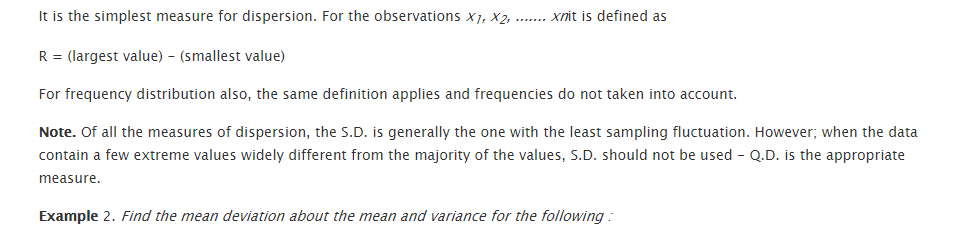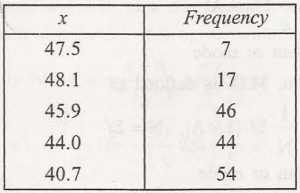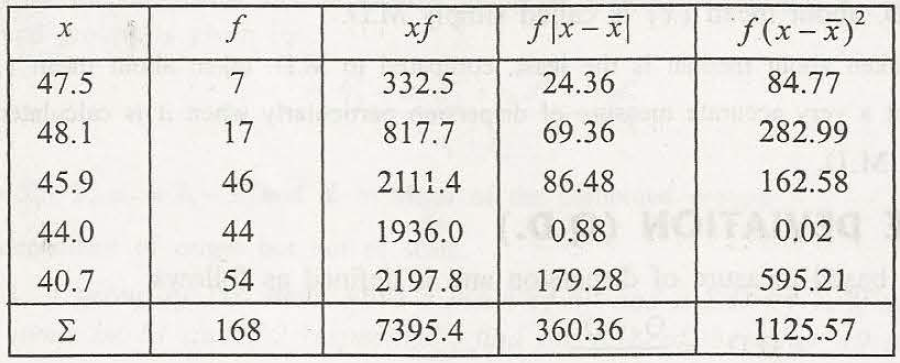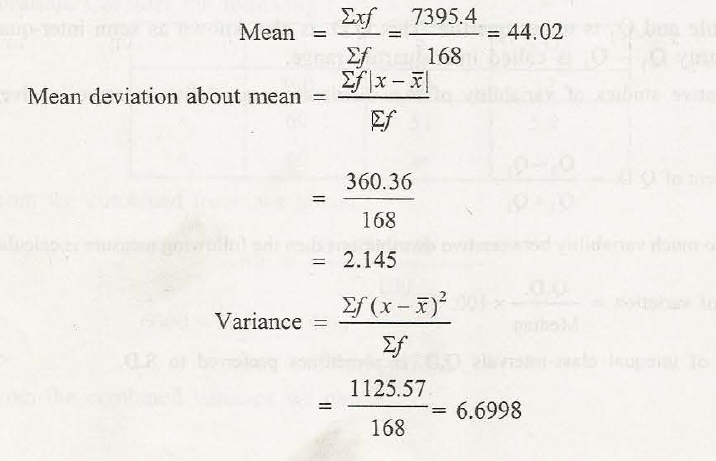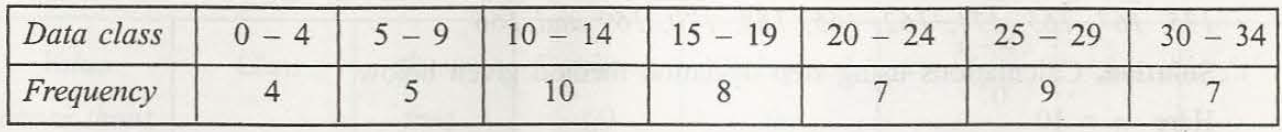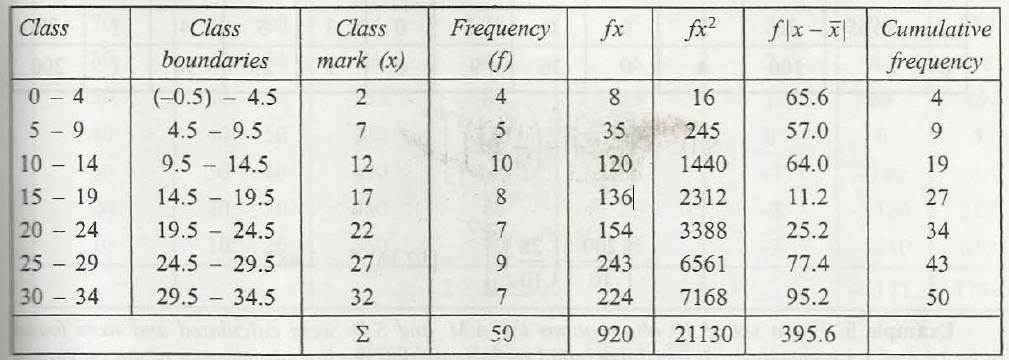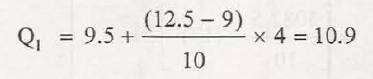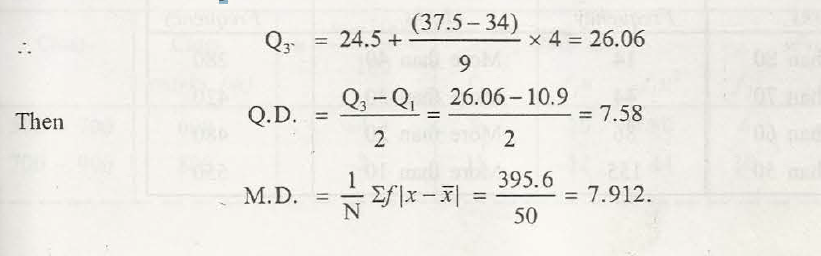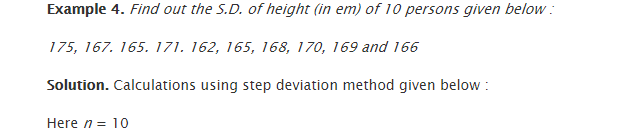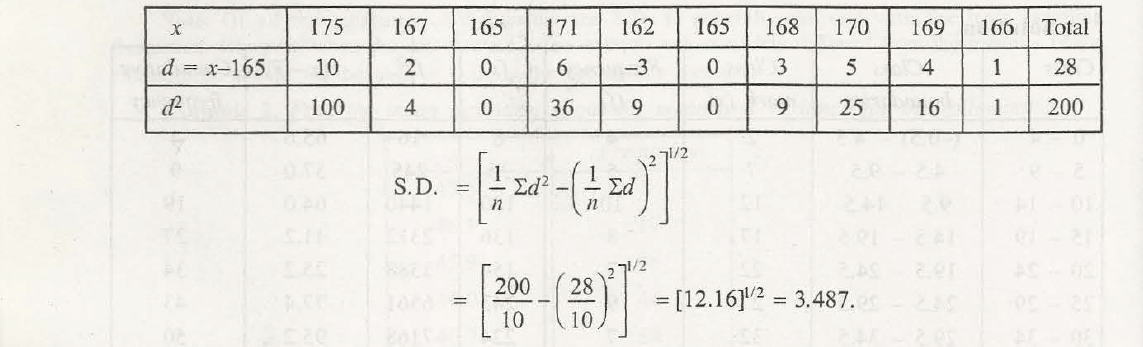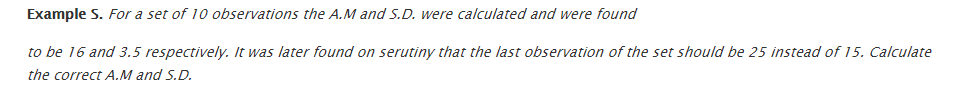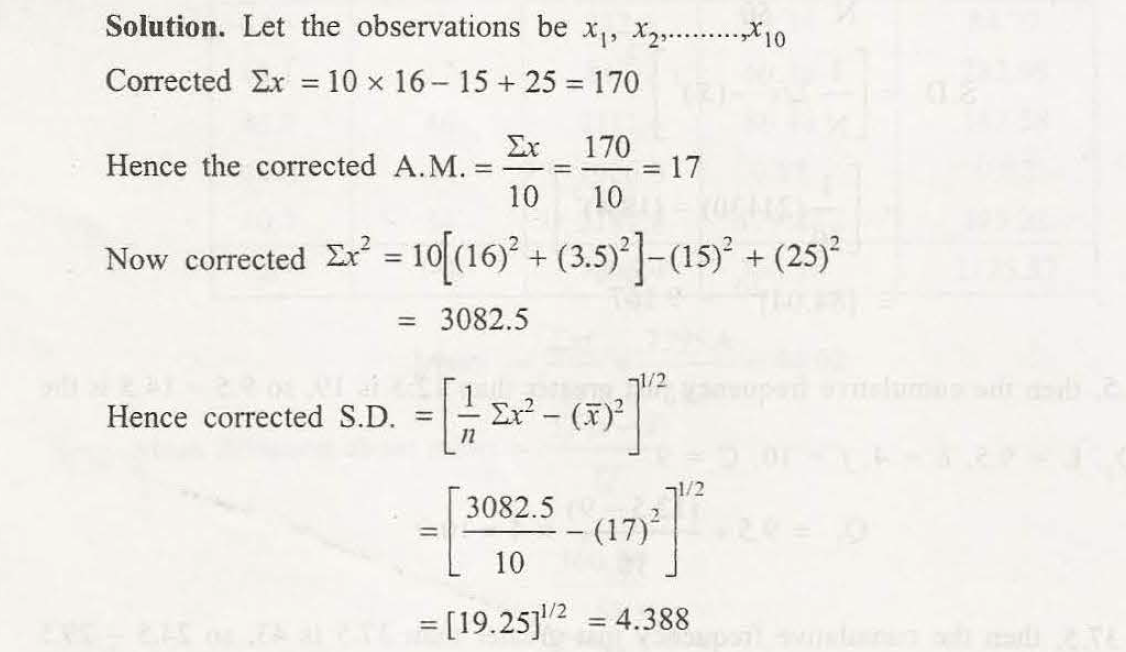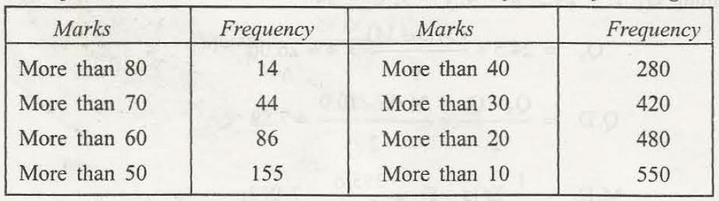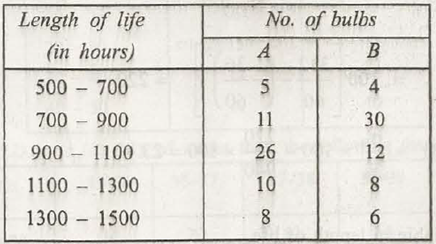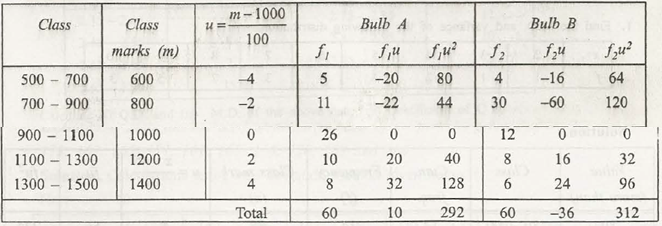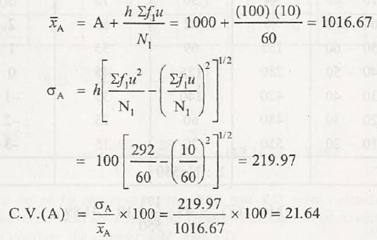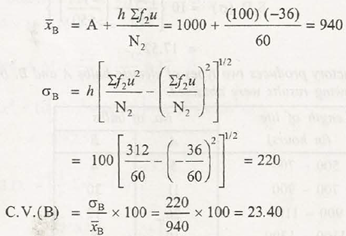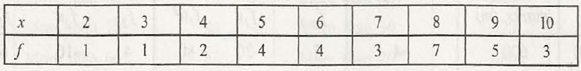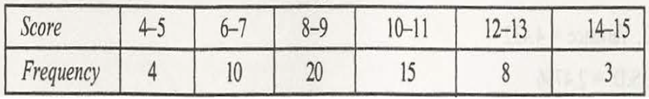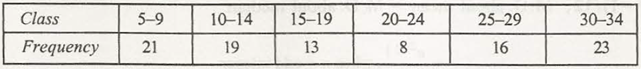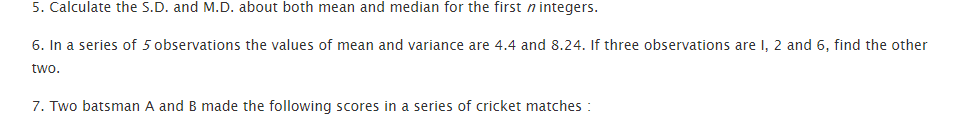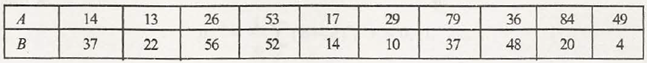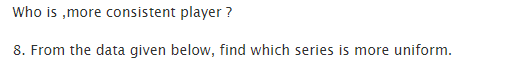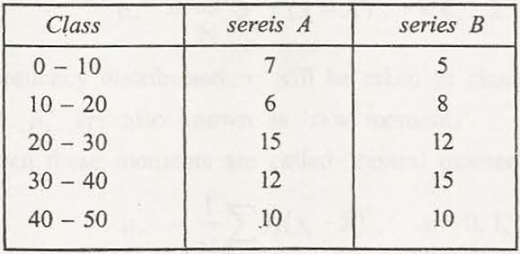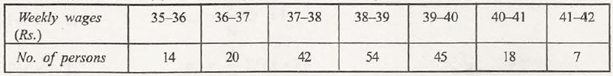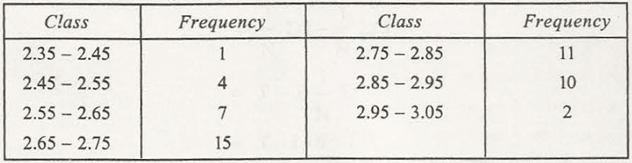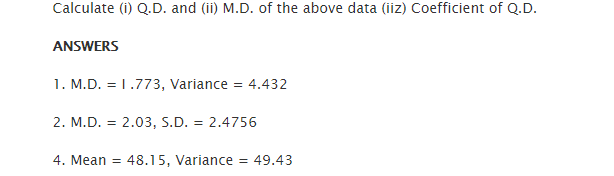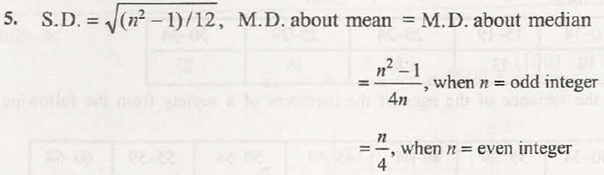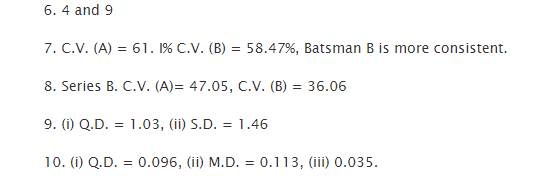### Customer Reviews

My Homework Help
Rated 5.0 out of 5 based on 510 customer reviews at